## Converting Units

### Learning Objective

1. Convert from one unit to another unit of the same type.

In Section 2.2 “Expressing Units”, we showed some examples of how to replace initial units with other units of the same type to get a numerical value that is easier to comprehend. In this section, we will formalize the process.

Consider a simple example: how many feet are there in 4 yards? Most people will almost automatically answer that there are 12 feet in 4 yards. How did you make this determination? Well, if there are 3 feet in 1 yard and there are 4 yards, then there are 4 × 3 = 12 feet in 4 yards.

This is correct, of course, but it is informal. Let us formalize it in a way that can be applied more generally. We know that 1 yard (yd) equals 3 feet (ft):

1 yd = 3 ft

In math, this expression is called an equality. The rules of algebra say that you can change (i.e., multiply or divide or add or subtract) the equality (as long as you don’t divide by zero) and the new expression will still be an equality. For example, if we divide both sides by 2, we get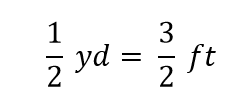We see that one-half of a yard equals 3/2, or one and a half, feet—something we also know to be true, so the above equation is still an equality. Going back to the original equality, suppose we divide both sides of the equation by 1 yard (number and unit):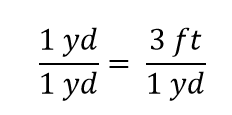The expression is still an equality, by the rules of algebra. The left fraction equals 1. It has the same quantity in the numerator and the denominator, so it must equal 1. The quantities in the numerator and denominator cancel, both the number and the unit: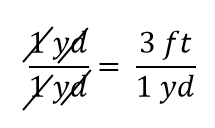When everything cancels in a fraction, the fraction reduces to 1: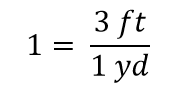We have an expression, 3 ft1 yd, that equals 1. This is a strange way to write 1, but it makes sense: 3 ft equal 1 yd, so the quantities in the numerator and denominator are the same quantity, just expressed with different units. The expression 3 ft1 yd is called a conversion factor, and it is used to formally change the unit of a quantity into another unit. (The process of converting units in such a formal fashion is sometimes called dimensional analysis or the factor label method.)

To see how this happens, let us start with the original quantity:

4 yd

Now let us multiply this quantity by 1. When you multiply anything by 1, you don’t change the value of the quantity. Rather than multiplying by just 1, let us write 1 as 3 ft1 yd: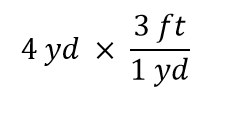The 4 yd term can be thought of as 4 yd/1; that is, it can be thought of as a fraction with 1 in the denominator. We are essentially multiplying fractions. If the same thing appears in the numerator and denominator of a fraction, they cancel. In this case, what cancels is the unit yard: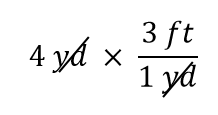That is all that we can cancel. Now, multiply and divide all the numbers to get the final answer: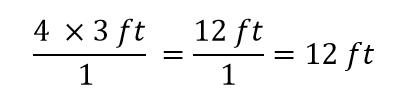Again, we get an answer of 12 ft, just as we did originally. But in this case, we used a more formal procedure that is applicable to a variety of problems.

How many millimeters are in 14.66 m? To answer this, we need to construct a conversion factor between millimeters and meters and apply it correctly to the original quantity. We start with the definition of a millimeter, which is

1 mm = 1/1,000 m

The 1/1,000 is what the prefix milli- means. Most people are more comfortable working without fractions, so we will rewrite this equation by bringing the 1,000 into the numerator of the other side of the equation:

1,000 mm = 1 m

Now we construct a conversion factor by dividing one quantity into both sides. But now a question arises: which quantity do we divide by? It turns out that we have two choices, and the two choices will give us different conversion factors, both of which equal 1: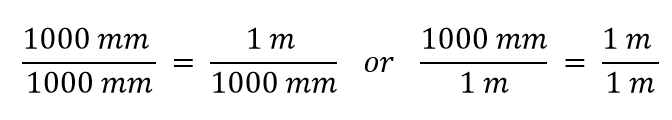Which conversion factor do we use? The answer is based on what unit you want to get rid of in your initial quantity. The original unit of our quantity is meters, which we want to convert to millimeters. Because the original unit is assumed to be in the numerator, to get rid of it, we want the meter unit in the denominator; then they will cancel. Therefore, we will use the second conversion factor. Canceling units and performing the mathematics, we get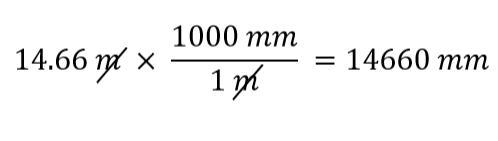Note how m cancels, leaving mm, which is the unit of interest.

The ability to construct and apply proper conversion factors is a very powerful mathematical technique in chemistry. You need to master this technique if you are going to be successful in this and future courses.

### Example 7

1. Convert 35.9 kL to liters.
2. Convert 555 nm to meters.

Solution

1. We will use the fact that 1 kL = 1,000 L. Of the two conversion factors that can be defined, the one that will work is 1,000 L/1 kL. Applying this conversion factor, we get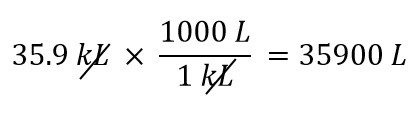2. We will use the fact that 1 nm = 1/1,000,000,000 m, which we will rewrite as 1,000,000,000 nm = 1 m, or 109 nm = 1 m. Of the two possible conversion factors, the appropriate one has the nm unit in the denominator: 1 m/109 nm. Applying this conversion factor, we get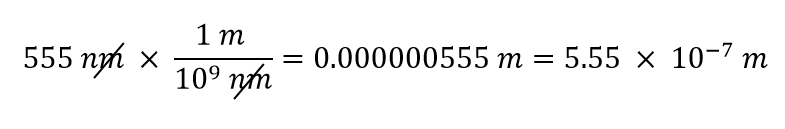In the final step, we expressed the answer in scientific notation.

Test Yourself

1. Convert 67.08 μL to liters.
2. Convert 56.8 m to kilometers.

1. 6.708 × 10−5 L
2. 5.68 × 10−2 km

What if we have a derived unit that is the product of more than one unit, such as m2? Suppose we want to convert square meters to square centimeters? The key is to remember that m2 means m × m, which means we have two meter units in our derived unit. That means we have to include two conversion factors, one for each unit. For example, to convert 17.6 m2 to square centimeters, we perform the conversion as follows: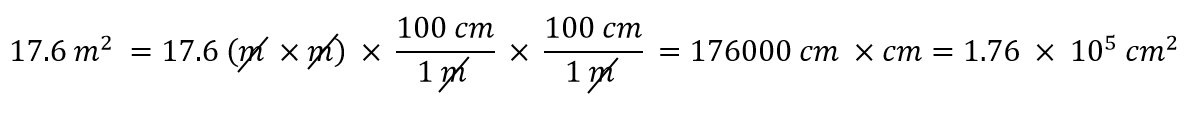### Example 8

How many cubic centimeters are in 0.883 m3?

Solution

With an exponent of 3, we have three length units, so by extension we need to use three conversion factors between meters and centimeters. Thus, we have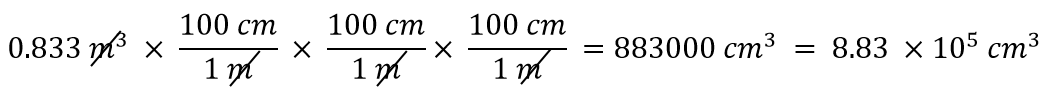You should demonstrate to yourself that the three meter units do indeed cancel.

Test Yourself

How many cubic millimeters are present in 0.0923 m3?

9.23 × 107 mm3

Suppose the unit you want to convert is in the denominator of a derived unit; what then? Then, in the conversion factor, the unit you want to remove must be in the numerator. This will cancel with the original unit in the denominator and introduce a new unit in the denominator. The following example illustrates this situation.

### Example 9

Convert 88.4 m/min to meters/second.

Solution

We want to change the unit in the denominator from minutes to seconds. Because there are 60 seconds in 1 minute (60 s = 1 min), we construct a conversion factor so that the unit we want to remove, minutes, is in the numerator: 1 min/60 s. Apply and perform the math: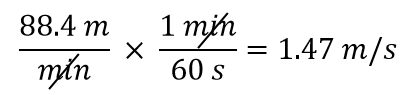Notice how the 88.4 automatically goes in the numerator. That’s because any number can be thought of as being in the numerator of a fraction divided by 1.

Test Yourself

Convert 0.203 m/min to meters/second.

0.00338 m/s or 3.38 × 10−3 m/s

Figure 2.8 How Fast Is Fast?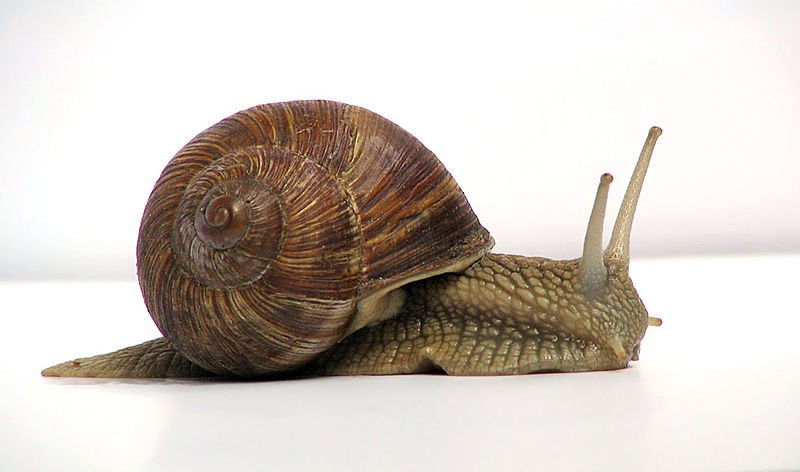A common garden snail moves at a rate of about 0.2 m/min, which is about 0.003 m/s, which is 3 mm/s!

Sometimes there will be a need to convert from one unit with one numerical prefix to another unit with a different numerical prefix. How do we handle those conversions? Well, you could memorize the conversion factors that interrelate all numerical prefixes. Or you can go the easier route: first convert the quantity to the base unit, the unit with no numerical prefix, using the definition of the original prefix. Then convert the quantity in the base unit to the desired unit using the definition of the second prefix. You can do the conversion in two separate steps or as one long algebraic step. For example, to convert 2.77 kg to milligrams:

Alternatively, it can be done in a single multistep process: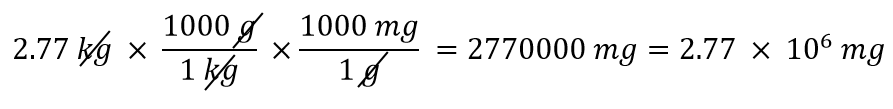You get the same answer either way.

### Example 10

How many nanoseconds are in 368.09 μs?

Solution

You can either do this as a one-step conversion from microseconds to nanoseconds or convert to the base unit first and then to the final desired unit. We will use the second method here, showing the two steps in a single line. Using the definitions of the prefixes micro- and nano-,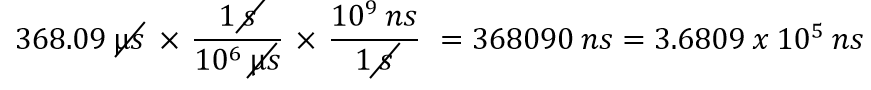Test Yourself

How many milliliters are in 607.8 kL?

6.078 × 108 mL

When considering the significant figures of a final numerical answer in a conversion, there is one important case where a number does not impact the number of significant figures in a final answer—the so-called exact number. An exact number is a number from a defined relationship, not a measured one. For example, the prefix kilo- means 1,000—exactly 1,000, no more or no less. Thus, in constructing the conversion factor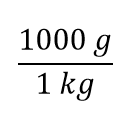neither the 1,000 nor the 1 enter into our consideration of significant figures. The numbers in the numerator and denominator are defined exactly by what the prefix kilo- means. Another way of thinking about it is that these numbers can be thought of as having an infinite number of significant figures, such as

The other numbers in the calculation will determine the number of significant figures in the final answer.

### Example 11

A rectangular plot in a garden has the dimensions 36.7 cm by 128.8 cm. What is the area of the garden plot in square meters? Express your answer in the proper number of significant figures.

Solution

Area is defined as the product of the two dimensions, which we then have to convert to square meters and express our final answer to the correct number of significant figures, which in this case will be three.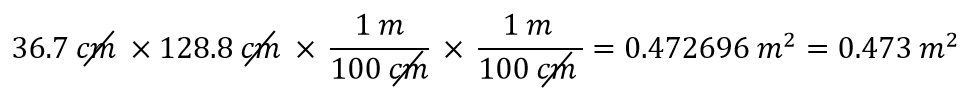The 1 and 100 in the conversion factors do not affect the determination of significant figures because they are exact numbers, defined by the centi- prefix.

Test Yourself

What is the volume of a block in cubic meters whose dimensions are 2.1 cm × 34.0 cm × 118 cm?

0.0084 m3

### Chemistry Is Everywhere: The Gimli Glider

On July 23, 1983, an Air Canada Boeing 767 jet had to glide to an emergency landing at Gimli Industrial Park Airport in Gimli, Manitoba, because it unexpectedly ran out of fuel during flight. There was no loss of life in the course of the emergency landing, only some minor injuries associated in part with the evacuation of the craft after landing. For the remainder of its operational life (the plane was retired in 2008), the aircraft was nicknamed “the Gimli Glider.”

The 767 took off from Montreal on its way to Ottawa, ultimately heading for Edmonton, Canada. About halfway through the flight, all the engines on the plane began to shut down because of a lack of fuel. When the final engine cut off, all electricity (which was generated by the engines) was lost; the plane became, essentially, a powerless glider. Captain Robert Pearson was an experienced glider pilot, although he had never flown a glider the size of a 767. First Officer Maurice Quintal quickly determined that the aircraft would not be able make it to Winnipeg, the next large airport. He suggested his old Royal Air Force base at Gimli Station, one of whose runways was still being used as a community airport. Between the efforts of the pilots and the flight crew, they managed to get the airplane safely on the ground (although with buckled landing gear) and all passengers off safely.

What happened? At the time, Canada was transitioning from the older English system to the metric system. The Boeing 767s were the first aircraft whose gauges were calibrated in the metric system of units (liters and kilograms) rather than the English system of units (gallons and pounds). Thus, when the fuel gauge read 22,300, the gauge meant kilograms, but the ground crew mistakenly fueled the plane with 22,300 pounds of fuel. This ended up being just less than half of the fuel needed to make the trip, causing the engines to quit about halfway to Ottawa. Quick thinking and extraordinary skill saved the lives of 61 passengers and 8 crew members—an incident that would not have occurred if people were watching their units.

Video source: Unit conversion by keyj (https://viuvideos.viu.ca/media/Unit+Conversion/0_o671v9j6)

### Key Takeaways

• Units can be converted to other units using the proper conversion factors.
• Conversion factors are constructed from equalities that relate two different units.
• Conversions can be a single step or multistep.
• Unit conversion is a powerful mathematical technique in chemistry that must be mastered.
• Exact numbers do not affect the determination of significant figures.

### Exercises

1. Write the two conversion factors that exist between the two given units.

a)  milliliters and liters

b)  microseconds and seconds

c)  kilometers and meters

2.  Write the two conversion factors that exist between the two given units.

a)  kilograms and grams

b)  milliseconds and seconds

c)  centimeters and meters

3.  Perform the following conversions.

a)  5.4 km to meters

b)  0.665 m to millimeters

c)  0.665 m to kilometers

4.  Perform the following conversions.

a)  90.6 mL to liters

b)  0.00066 ML to liters

c)  750 L to kiloliters

5.  Perform the following conversions.

a)  17.8 μg to grams

b)  7.22 × 102 kg to grams

c)  0.00118 g to nanograms

6.  Perform the following conversions.

a)  833 ns to seconds

b)  5.809 s to milliseconds

c)  2.77 × 106 s to megaseconds

7.  Perform the following conversions.

a)  9.44 m2 to square centimeters

b)  3.44 × 108 mm3 to cubic meters

8.  Perform the following conversions.

a)  0.00444 cm3 to cubic meters

b)  8.11 × 102 m2 to square nanometers

9.  Why would it be inappropriate to convert square centimeters to cubic meters?

10.  Why would it be inappropriate to convert from cubic meters to cubic seconds?

11.  Perform the following conversions.

a)  45.0 m/min to meters/second

b)  0.000444 m/s to micrometers/second

c)  60.0 km/h to kilometers/second

12.  Perform the following conversions.

a)  3.4 × 102 cm/s to centimeters/minute

b)  26.6 mm/s to millimeters/hour

c)  13.7 kg/L to kilograms/milliliters

13.  Perform the following conversions.

a)  0.674 kL to milliliters

b)  2.81 × 1012 mm to kilometers

c)  94.5 kg to milligrams

14.  Perform the following conversions.

a)  6.79 × 10−6 kg to micrograms

b)  1.22 mL to kiloliters

c)  9.508 × 10−9 ks to milliseconds

15.  Perform the following conversions.

a)  6.77 × 1014 ms to kiloseconds

b)  34,550,000 cm to kilometers

16.  Perform the following conversions.

a)  4.701 × 1015 mL to kiloliters

b)  8.022 × 10−11 ks to microseconds

17.  Perform the following conversions. Note that you will have to convert units in both the numerator and the denominator.

a)  88 ft/s to miles/hour (Hint: use 5,280 ft = 1 mi.)

b)  0.00667 km/h to meters/second

18.  Perform the following conversions. Note that you will have to convert units in both the numerator and the denominator.

a)  3.88 × 102 mm/s to kilometers/hour

b)  1.004 kg/L to grams/milliliter

19.  What is the area in square millimeters of a rectangle whose sides are 2.44 cm × 6.077 cm? Express the answer to the proper number of significant figures.

20.  What is the volume in cubic centimeters of a cube with sides of 0.774 m? Express the answer to the proper number of significant figures.

21.  The formula for the area of a triangle is 1/2 × base × height. What is the area of a triangle in square centimeters if its base is 1.007 m and its height is 0.665 m? Express the answer to the proper number of significant figures.

22.  The formula for the area of a triangle is 1/2 × base × height. What is the area of a triangle in square meters if its base is 166 mm and its height is 930.0 mm? Express the answer to the proper number of significant figures.

1.

a)  1,000 mL/1 L and 1 L/1,000 mL

b)  1,000,000 μs/1 s and 1 s/1,000,000 μs

c)  1,000 m/1 km and 1 km1,000 m

3.

a)  5,400 m

b)  665 mm

c)  6.65 × 10−4 km

5.

a)  1.78 × 10−5 g

b)  7.22 × 105 g

c) 1.18 × 106 ng

7.

a)  94,400 cm2

b)  0.344 m3

9.

One is a unit of area, and the other is a unit of volume.

11.

a)  0.75 m/s

b)  444 µm/s

c)  1.666 × 10−2 km/s

13.

a)  674,000 mL

b)  2.81 × 106 km

c)  9.45 × 107 mg

15.

a)  6.77 × 108 ks

b)  345.5 km

17.

a)  6.0 × 101 mi/h

b)  0.00185 m/s

19.

1.48 × 103 mm2

21.

3.35 × 103 cm2